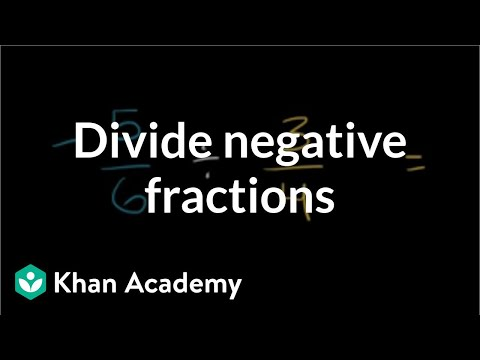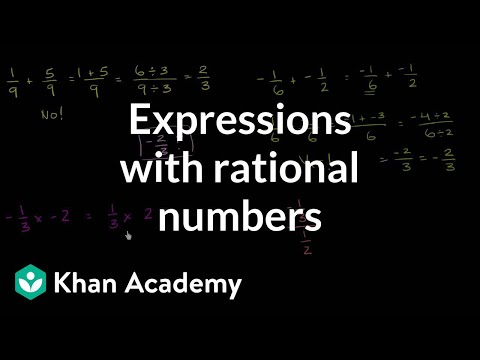Video

# Why a negative times a negative makes sense (Full video)

Description: Use the repeated addition model of multiplication to give an understanding of multiplying negative numbers. Created by Sal Khan. this deal does not make complete concrete sense to you, you want to have a slightly deeper institution than just having to accept its consistent with the distributive property and whatever else and so you try another thought experiment, you say "well what is just a basic multiplication way of doing it?" So if I say, two times three, one way to to conceptualize is basic multiplication is really repeating addition, so you could view this as two threes so let me write three plus three and notice there are two of them, there are two of these or you could view this as three twos, and so this is the same thing as two plus two plus two and there are three of them, and either way you can conceptualize as you get the same exact answer. Let's do two times negative three, I want to make the negative into a different color.

### Other videos you might be interested in### Dividing negative fractions (Full video)